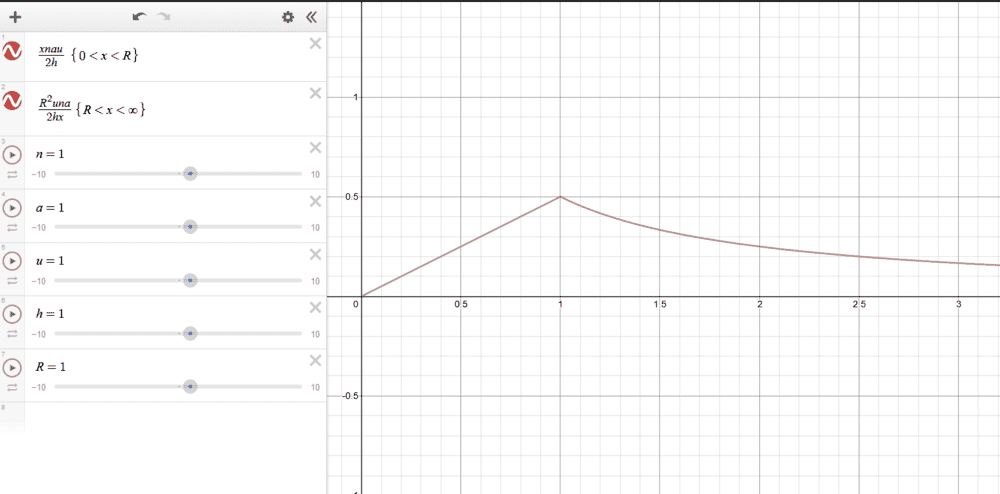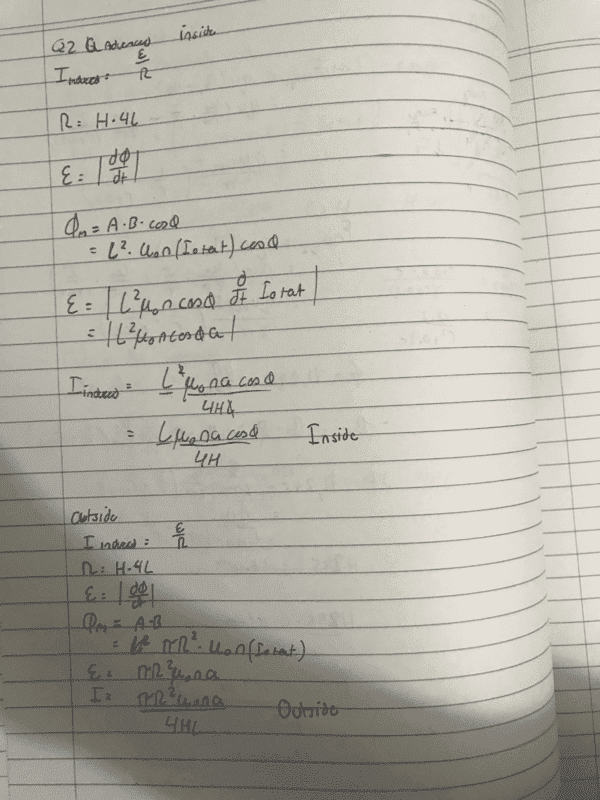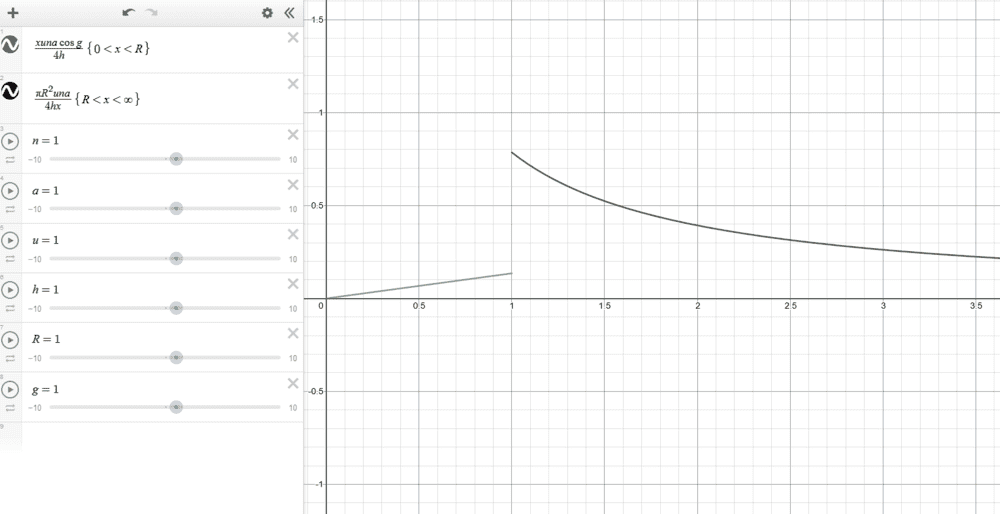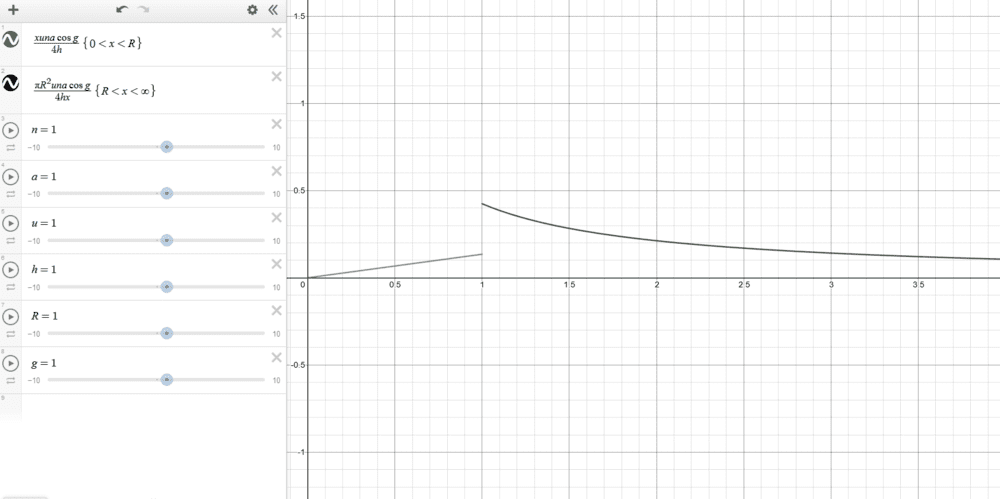# Induced Current In a Tilted Square Inside and Outside a Solenoid

• Cactus
So in this case, regardless of the angle between the square and the solenoid field, there would be no flux through the square.

#### Cactus

Homework Statement
Hey, so I've been given a question to find the induced current in a conducting square within a solenoid. The solenoid has a current running that is increasing so that the magnetic flux is increasing. The question first asked for a ring inside and outside the solenoid (encompassing it), for which I derived the two formulas in red on the graph (Where initially when the ring is inside the solenoid, the current induced constantly increases, however when the radius of the ring exceeds that of the solenoid it decreases at a rapid rate (as expected). However, when I apply the same working to the square, the plotted graph does not look right

These are the details and questions:
An ideal solenoid, of radius R and n turns per unit length, has a current flowing through it. The current, I, varies with time, t, according to I=I0+at where I0 and a are constants. A conducting ring of radius, r, is placed inside the solenoid with its axis coinciding with the axis of the solenoid. The ring has a resistance per unit length of H(in units of Ω/m).

Make a plot of the current in the ring as a function of the radius of the ring. Include curves for r < R and r > R. Explain what the plot shows. (Done Below)

The ring is replaced by a conducting wire in the shape of a square. The side length of the square is l, and it is made of the same conducting material as the ring. The square is tilted by an angle, θ (the angle between the area vector for the solenoid and the area vector for the square is θ). Repeat your calculations in Part A to determine the current in the square. Include calculations for when the square is completely inside the solenoid and completely outside the solenoid only

I am just wondering firstly if when both the ring and square encompass the solenoid, the correct area to use for the flux is that of the solenoids area as both r and l are greater than R. Likewise, are the following two formulas correct for the square, or should I include a cosθ for the outside (although the angle should not matter as all flux occurs in the solenoids cross sectional area, and even if I do include a cosθ the graphs still do not connect).
Relevant Equations
Current = emf/resistance
Solenoid Field = u*n*I (n = no. turns per unit length)
Flux = A*B*cosθ
Current in Solenoid = I0 + at (a is some constant)
Resistance = H * length or circumferencePlot for the ring ^Calculations for the Square ^Plot for square without cosg on the outside calc ^Plot for square with cosg on the outside calc ^

As can be seen the formulas for the square conductor do not connect at R, which I'm not sure if they should or if they should not as in this case the square does not fit the shape of the solenoid so the crossover occurs later (at the intersection of the two graphs) (whilst the ring r can equal R as they are both circles). Or otherwise, I am not sure if my formula derived is correct

It is good that you are worried. In your equation for Flux, carefully define each component for me.

hutchphd said:
It is good that you are worried. In your equation for Flux, carefully define each component for me.
For each equation all variables are as follows:
L = side length of square
μ0 = permeability constant (4pi*10^-7)
n = number of turns per unit length of the solenoid
a = constant from the current formula I(solenoid) = I0 + at
cosθ = angle between the area vector of the square and of the solenoid field (In the desmos graph I replaced theta with g)
H = resistance per unit length of the conducting object
R = Radius of the solenoid
r = radius of the ring
A = Area
B = Magnetic Field
R = Resistance
ε = emf/voltage
I = Current in the conducting object (ring or square)

Yes but Area of what?

hutchphd said:
Yes but Area of what?
Area as in the area of which the magnetic field lines pass through, so for the ring inside the solenoid the field lines would pass through an area of pi*r^2 as all of the 'area' of the ring is inside the solenoid and thus in the magnetic field whereas when the ring radius is greater than the solenoid the only area the field lines are passing through is pi*R^2 as the circle formed by the solenoid is smaller than that formed by the ring but encompassed by the ring

Your problem here is one of geometry. For the square (tilted or not) their is clearly an intermediate size region where it is neither completely inside nor outside. You need to figure out the "flux linkage area" to find the flux.

I see what you're getting at. I would assume in this case my calculations would be correct for when the square is completely outside and completely inside (or would I need to still consider the angle between the square area vector and the field lines when the square is outside). In that case I shouldn't need to worry about the region in which it is both inside and outside as the question asks for only when the square is completely inside or completely outside. Thus in the graph the point of discontinuity occurs due to that region where it is both inside and outside which I am not required to calculate for?

Yes the angle will matter inside. I didn't comprehend that you can ignore the "both" region (it seemed like a pretty nasty thing to solve leading to transcendental expressions I expect!). But there is no discontinuous change.
So you seem on top of this I think..although the graphs are tough to read in detail.

Last edited:
hutchphd said:
Yes the angle will matter inside. I didn't comprehend that you can ignore the "both" region (it seemed like a pretty nasty thing to solve leading to transcendental expressions I expect!). But there is no discontinuous change.
So you seem on top of this I think..although the graphs are tough to read in detail.

Thanks for pointing out that fact of the square being both inside and outside, I didn't realize that was what would be most likely causing the discontinuity until you pointed it out.
Also one last question if you don't mind me asking
I understand that when the square is inside the solenoid, the angle between the vectors will play a part, however when the square is entirely outside the solenoid would this angle still play a role as regardless of the square being at an angle or not an equal amount of flux lines would be passing through the square?

For an "infinite" solenoid where the field is ~zero outside, no allowed deformation of the loop will matter because you pass through no field lines.